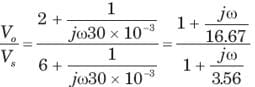Test: Frequency Response

# Test: Frequency Response - Electrical Engineering (EE)

Test Description

## 20 Questions MCQ Test GATE Electrical Engineering (EE) 2024 Mock Test Series - Test: Frequency Response

Test: Frequency Response for Electrical Engineering (EE) 2023 is part of GATE Electrical Engineering (EE) 2024 Mock Test Series preparation. The Test: Frequency Response questions and answers have been prepared according to the Electrical Engineering (EE) exam syllabus.The Test: Frequency Response MCQs are made for Electrical Engineering (EE) 2023 Exam. Find important definitions, questions, notes, meanings, examples, exercises, MCQs and online tests for Test: Frequency Response below.
Solutions of Test: Frequency Response questions in English are available as part of our GATE Electrical Engineering (EE) 2024 Mock Test Series for Electrical Engineering (EE) & Test: Frequency Response solutions in Hindi for GATE Electrical Engineering (EE) 2024 Mock Test Series course. Download more important topics, notes, lectures and mock test series for Electrical Engineering (EE) Exam by signing up for free. Attempt Test: Frequency Response | 20 questions in 60 minutes | Mock test for Electrical Engineering (EE) preparation | Free important questions MCQ to study GATE Electrical Engineering (EE) 2024 Mock Test Series for Electrical Engineering (EE) Exam | Download free PDF with solutions
 1 Crore+ students have signed up on EduRev. Have you?
Test: Frequency Response - Question 1

### A parallel resonant circuit has a resistance of 2 kΩ and half power frequencies of 86 kHz and 90 kHz.1. The value of capacitor is

Detailed Solution for Test: Frequency Response - Question 1

BW = ω2 - ω1 = 2π(90 - 86)k = 8π krad/s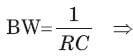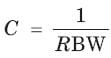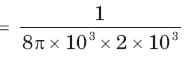= 19.89 nF

Test: Frequency Response - Question 2

### A parallel resonant circuit has a resistance of 2 kΩ and half power frequencies of 86 kHz and 90 kHz.The value of inductor is

Detailed Solution for Test: Frequency Response - Question 2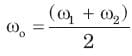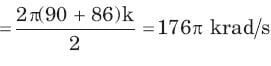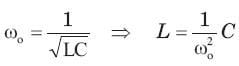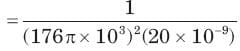= 0.16 mH

Test: Frequency Response - Question 3

### A parallel resonant circuit has a resistance of 2 kΩ and half power frequencies of 86 kHz and 90 kHz.The quality factor is

Detailed Solution for Test: Frequency Response - Question 3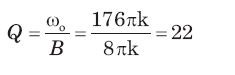Test: Frequency Response - Question 4

A parallel resonant circuit has a midband admittance of 25 x 10-3 S, quality factor of 80 and a resonant frequency of 200 krad/s.The value of R is

Detailed Solution for Test: Frequency Response - Question 4

Ay mid-band frequency Z = R, Y = 1/R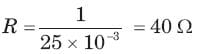Test: Frequency Response - Question 5

A parallel resonant circuit has a midband admittance of 25 x 10-3 S, quality factor of 80 and a resonant frequency of 200 krad/s.The value of C is

Detailed Solution for Test: Frequency Response - Question 5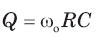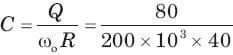= 10 μF

Test: Frequency Response - Question 6

A parallel RLC circuit has R =1 kΩ and C = 1 μF. Thequality factor at resonance is 200. The value of inductor is

Detailed Solution for Test: Frequency Response - Question 6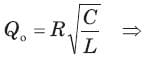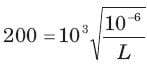⇒ L = 25 μH

Test: Frequency Response - Question 7

A parallel circuit has R =1 k , C = 50 μF and L = 10mH. The quality factor at resonance is

Detailed Solution for Test: Frequency Response - Question 7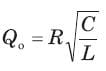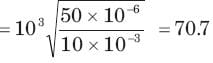Test: Frequency Response - Question 8

A series resonant circuit has an inductor L = 10 mH.The resonant frequency ωo = 106 rad/s and bandwidth is BW = 103 rad/s. The value of R and C will be

Detailed Solution for Test: Frequency Response - Question 8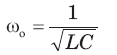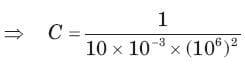= 100pF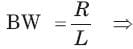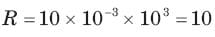Test: Frequency Response - Question 9

A series resonant circuit has L = 1 mH and C = 10μF. The required R for the BW 15 9 . Hz is

Detailed Solution for Test: Frequency Response - Question 9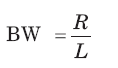⇒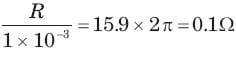Test: Frequency Response - Question 10

For the RLC parallel resonant circuit when R = 8 kΩ , L = 40 mH and C = 0.25 μF, the quality factor Q is

Detailed Solution for Test: Frequency Response - Question 10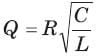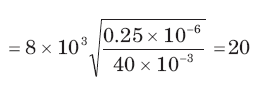Test: Frequency Response - Question 11

The maximum voltage across capacitor would be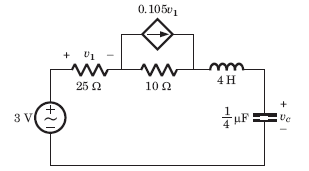Detailed Solution for Test: Frequency Response - Question 11

Thevenin equivalent seen by L - C combination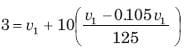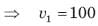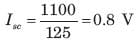Open Circuit : v1 = 0, v∝ = 3V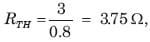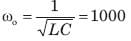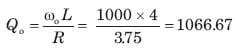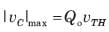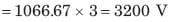Test: Frequency Response - Question 12

For the circuit shown in fig. resonant frequency fo is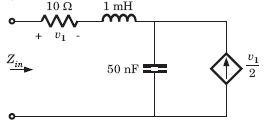Detailed Solution for Test: Frequency Response - Question 12

Applying 1 A at input port V1 = 10 V
voltage across 1 A source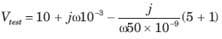Zin = Vtest
At resonance Im {Zin} = 0
⇒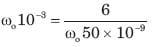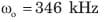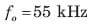Test: Frequency Response - Question 13

For the circuit shown in fig. the resonant frequency fo is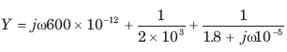Detailed Solution for Test: Frequency Response - Question 13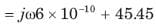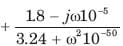At resonance Im {Y} = 0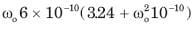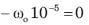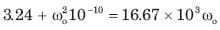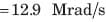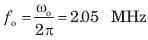Test: Frequency Response - Question 14

The network function of circuit shown in fig. is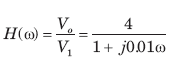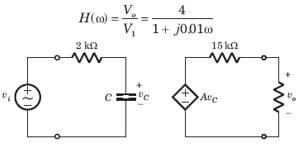Detailed Solution for Test: Frequency Response - Question 14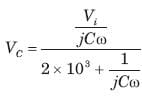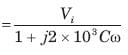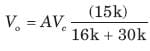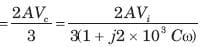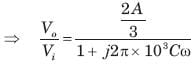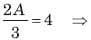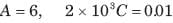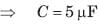Test: Frequency Response - Question 15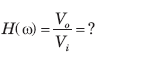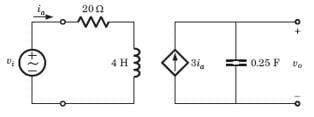Detailed Solution for Test: Frequency Response - Question 15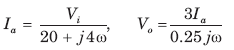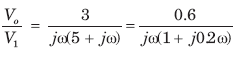Test: Frequency Response - Question 16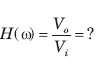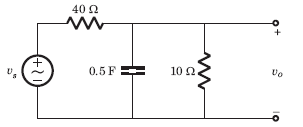Detailed Solution for Test: Frequency Response - Question 16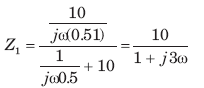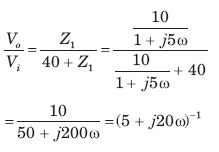Test: Frequency Response - Question 17

The value of input frequency is required to cause again equal to 1.5. The value is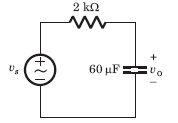Detailed Solution for Test: Frequency Response - Question 17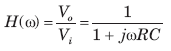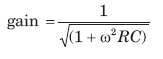For any value of ω, R,C gain ≤ 1.
Thus (D) is correct option.

Test: Frequency Response - Question 18

In the circuit of fig. phase shift equal to -450 is required at frequency ω =20 rad/s . The value of R is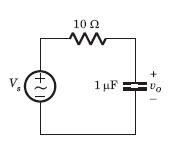Detailed Solution for Test: Frequency Response - Question 18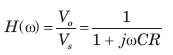phase shift = - tan-1 ωCR = -450°
ωCR = 1,

20 x 1 x 10-6 R = 1 ⇒ R = 50KΩ

Test: Frequency Response - Question 19

For the circuit of fig., Vs is the source voltage and the response is the resistance voltage Vo, R = 30Ω and L = 2H. Suppose the input frequency is adjusted until the gain is equal to 0.6. The value of the frequency is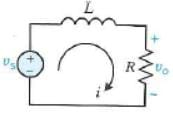Detailed Solution for Test: Frequency Response - Question 19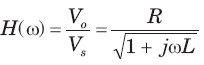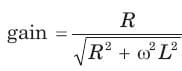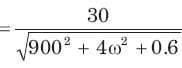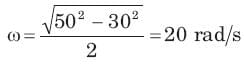Test: Frequency Response - Question 20

Bode diagram of the network function Vo /Vfor the circuit of fig.  is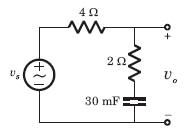Detailed Solution for Test: Frequency Response - Question 20